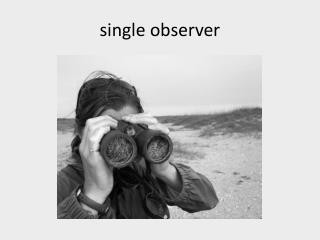DownloadDownload Presentationsingle observer

# single observer

Télécharger la présentation## single observer

- - - - - - - - - - - - - - - - - - - - - - - - - - - E N D - - - - - - - - - - - - - - - - - - - - - - - - - - -
##### Presentation Transcript

1. single observer

2. multiple observers • independent observers • observers sample same locations simultaneously and independently • match observations when the point count is over • matched observations, together with observations unique to each observer, provide information about each observers unique detection probability • Pd only

3. multiple observers N observer 1 observer 2 …after matching

4. detection probability the probability of detection for each observer is p1 = m2/n2 and p2 = m2/n1 where: p is detection probability m is the number of birds seen by both observers ni is the number seen by one observer i

5. population size The population size can then be estimated as follows: N = (n1*n2)/m2 where: N is population size m is the number of birds seen by both observers ni is the number seen by one observer i

6. Multiple Observers • Critical Assumptions • Observers do not influence each other’s detections • No matching errors • The sample area is closed to bird movements • For each observer, individual birds are not double-counted and multiple birds are not lumped into one